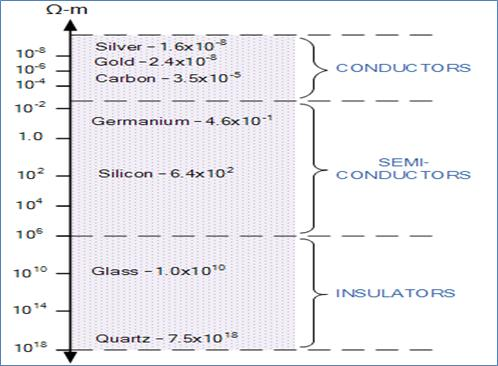Loading, please wait...## Resistor

Resistivity/Resistance

According to Ohm’s law, the electrical Resistance is defined as the ratio of the voltage difference across the system to the current flowing through it. Formulae of resistivity are defined as:

Where:R is the electrical resistance of a uniform specimen of the material measured in ohms
l is the length of the piece of material measured in meters, m
A is the cross-sectional area of the specimen measured in square meters, m2

The resistance varies accordingly the physical size of the material being measured as well as the material from which it is constructed. For example, if the

length of the material increases its resistance would increase proportionally.

Similarly, if we increased its diameter or size (making it thicker) its resistance value would decrease If the resistivity of various materials is compared, they can be classified into three main groups, Conductors, Insulators and Semi-conductors as shown below.